# Texas Go Math Grade 6 Lesson 8.4 Answer Key Converting Measurements

Refer to our Texas Go Math Grade 6 Answer Key Pdf to score good marks in the exams. Test yourself by practicing the problems from Texas Go Math Grade 6 Lesson 8.4 Answer Key Solving Converting Measurements.

## Texas Go Math Grade 6 Lesson 8.4 Answer Key Converting Measurements

Reflect

Question 1.
Communicate Mathematical Ideas How could you draw a model to show the relationship between feet and inches?
1 feet = 12 inches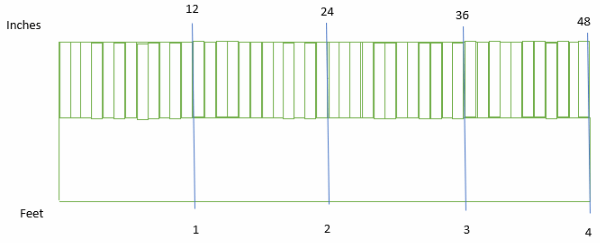Question 2.
The height of a doorway is 2 yards. What is the height of the doorway in inches?Question 3.
An oak tree is planted when it is 250 centimeters tall. What ¡s this height in meters?
1 gallon = 16 cups
$$\frac{\text { gallon }}{\text { cups }}=\frac{1}{16}=\frac{2}{x}$$
2 is a common numerator:
$$\frac{1}{16} \cdot \frac{2}{2}=\frac{2}{x}$$
$$\frac{2}{32}=\frac{2}{x}$$
⇒ x = 32
32 cups of punch

Use the model below to complete each statement.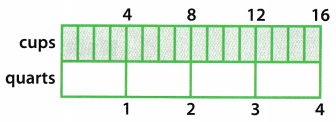Question 1.
$$\frac{4}{1}=\frac{12}{3}$$, so 12 cups = __________ quarts
4 cups make up 1 quart, therefore 12 cups will make $$\frac{12}{4}$$ = 3 quarts.
12 cups= 3 quarts.

Question 2.
$$\frac{4}{1}=\frac{48}{12}$$, so __________ cups = 12 quarts
4 cups make up 1 quart, therefore 12 quart will contain 12 × 4 = 48 cups.
12 quarts = 48 cups.

Use unit rates to solve.

Question 3.
Mary Catherine makes 2 gallons of punch for her party. How many cups of punch did she make?
1 gallon is equal to 16 cups so 2 gallons will be equal to 2 × 16 = 32 cups. Therefore, Mary Catherine made 32 cups of punch for her party.

Mary Catherine made 32 cups of punch for her party.

Question 4.
An African elephant weighs 6 tons. What is the weight of the elephant in pounds?
1 ton is equal to 2000 pounds so 6 tons will be equal to 6 × 2000 = 12000 pounds. Therefore, the weight of the elephant is 12000 pounds.

The weight of the elephant is 12000 pounds.

Question 5.
The distance from Jason’s house to school is 0.5 kilometer. What is this distance in meters?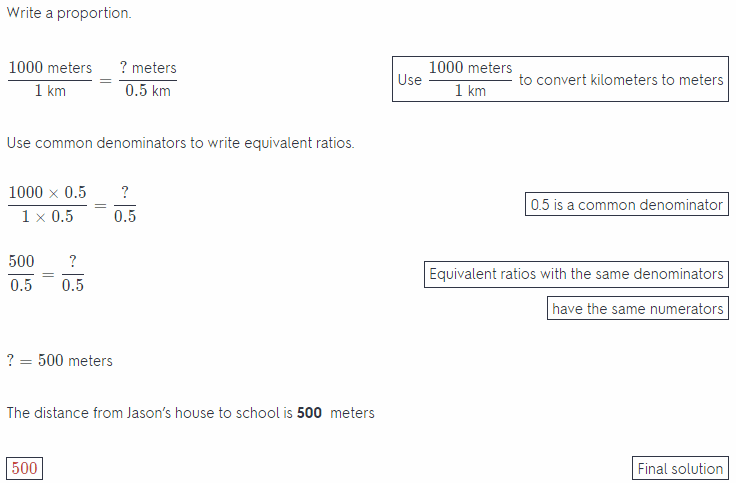Question 6.
The mass of a moon rock is 3.5 kilograms. What is the mass of the moon rock in grams?Use a conversion factor to solve.

Question 7.
1.75 grams ∙ $$\frac{1,000 \mathrm{mg}}{1 \mathrm{~g}}$$ = _____________
Find the conversion factor.
Write 1000 miligrams = 1 grams as a ratio: $$\frac{1000 \text { miligrams }}{1 \text { grams }}$$
Multiply the given measurement by the conversion factor
1.75 grams ∙ $$\frac{1000 \mathrm{mg}}{1 \mathrm{~g}}$$ = ? mg
1.75 grams ∙ $$\frac{1000 \mathrm{mg}}{1 \mathrm{~g}}$$ = 1750 mg [Cancel the common unit]
1750 = Final solution

Question 8.
27 millimeters ∙ $$\frac{1 \mathrm{~cm}}{10 \mathrm{~mm}}$$ = _____________
Find the conversion factor
Write 1 cm t o mm: $$\frac{1 \mathrm{~cm}}{10 \mathrm{~mm}}$$
Multiply the given measurement by the conversion factor
27 mm $$\frac{1 \mathrm{~cm}}{10 \mathrm{~mm}}$$ = ? cm
27 mm $$\frac{1 \mathrm{~cm}}{10 \mathrm{~mm}}$$ = 2.7 cm [Cancel the common unit]
2.7 = Final solution

Question 9.
A package weighs 96 ounces. What is the weight of the package in pounds?
Find the conversion factor
Write 1 pound = 16 ounces as a ratio: $$\frac{1 \text { pound }}{16 \text { ounces }}$$
Multiply the given measurement by the conversion factor
96 ounces $$\frac{1 \text { pound }}{16 \text { ounces }}$$ = ? pounds
96 ounces $$\frac{1 \text { pound }}{16 \text { ounces }}$$ = 16 pounds [Cancel the common unit]
The weight of the package is 6 pounds
6 = Final, solution

Question 10.
A jet flies at an altitude of 52,800 feet. What is the height of the jet in miles?
Find the conversion factor
Write 1 mile 5280 feet as a ratio: $$\frac{1 \text { mile }}{5280 \text { feet }}$$
Multiply the given measurement by the conversion factor
52800 feet $$\frac{1 \text { mile }}{5280 \text { feet }}$$ = ? miles
52800 feet $$\frac{1 \text { mile }}{5280 \text { feet }}$$ = 10 miles [Cancel the common unit]
The height of the jet is 10 miles
10 = Final solution

Essential Question Check-In

Question 11.
How do you convert units within a measurement system?
Units are inter changeable among each other by the constant of conversion. For example 1 meter is equal to 100 centimeters, so here 100 is the constant of conversion. Any length in meters is converted to an equivalent length in centimeters by multiplying it with 100. Similarly, any length in centimeters is converted to an equivalent length in meters by dividing it with 100.

Question 12.
What is a conversion factor that you can use to convert gallons to pints? How did you find it?
We know that 1 quart is equal to 2 pints and 1 gallon is equal to 4 quarts, so it can be calculated that 1 gallon is equal to 2 × 4 = 8 pints.

1 gallon is equal to 8 pints.

Question 13.
Three friends each have some ribbon. Carol has 42 inches of ribbon, Tino has 2.5 feet of ribbon, and Baxter has 1.5 yards of ribbon. Express the total length of ribbon the three friends have in inches, feet and yards. ____ inches = ____ feet = ____ yards
Carol has 42 inches of ribbon, Tino has 25 feet of ribbon, and Baxter has 15 yards of ribbon. First convert all Lengths to inches, therefore Tino has 2.5 × 12 = 30 inches of ribbon and Baxter has 1.5 × 3 × 12 = 54 inches of ribbon. Total Length in inches is 42 + 30 + 54 = 126.

Divide this length by 12 to convert it to an equivalent length in feet, therefore 126 inches = $$\frac{126}{12}$$ = 10.5 feet.

Divide this length by 3 to convert it to an equivalent length in yards, therefore 10.5 inches = $$\frac{10.5}{3}$$ = 3.5 feet.

126 inches = 10.5 feet = 3.5 yards.

Question 14.
Suzanna wants to measure a board, but she doesn’t have a ruler to measure with. However, she does have several copies of a book that she knows is 17 centimeters tall.

a. Suzanna lays the books end to end and finds that the board is the same length as 21 books. How many centimeters long is the board?
The board is the same length as 21 books and the length of the book is 17 centimeters, therefore, the board is 21 × 17 = 357 centimeters long.

b. Suzanna needs a board that is at least 3.5 meters long, Is the board long enough? Explain.
357 centimeters can be converted to an equivalent length in meters by dividing by 100, therefore 357 centimeters is equal to $$\frac{357}{100}$$ = 3.57 meters. This is greater than the minimum length of board required, so this board is long enough for her use.

Sheldon needs to buy 8 gallons of ice cream for a family reunion. The table shows the prices for different sizes of two brands of ice cream.Question 15.
Which size container of Cold Farm ice cream ¡s the better deal for Sheldon? Explain.
2 of the 1 options shown display die rate in dollars per quart, so convert the remaining 2 rates in dollars per quart. There are 2 pints in 1 quart, so 1 pint is equal to $$\frac{1}{2}$$ = 0.5 pints. This implies that the rate of ice cream at $2.5 per pint is actually $$\frac{\ 2.5}{0.5}$$ =$5 per quart. Therefore. $4.50 for 1 quart is a better option here. Question 16. Multistep Which size and brand of ice cream is the best deal? Answer: 2 of the 4 options shown display the rate in dollars per quart, so convert the remaining 2 rates in dollars per quart. There are 2 pints in 1 quart, so 1 pint is equal to $$\frac{1}{2}$$ = 0.5 pints. This implies that the rate of ice cream at$ 2.5 per pint is actually $$\frac{\ 2.5}{0.5}$$ = $5 per quart. Step 2 Also, There are i quarts in 1 gallon. This implies that the rate of ice cream at$9.5 per gallon is actually $$\frac{\ 9.5}{4}$$ = $2.375 per quart. Step 3 The$9.50 for 1 gallon from Cone Dreams is the most economicaL option here and so the best deal.

Question 17.
In Beijing in 2008, the Women’s 3,000 meter Steeplechase became an Olympic event. What is this distance in kilometers? ________________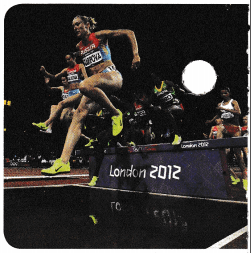Find the conversion factor
Write 1 km = 1000 meters as a ratio: $$\frac{1 \mathrm{~km}}{1000 \text { meters }}$$
Multiply the given measurement by the conversion factor
3000 meters ∙ $$\frac{1 \mathrm{~km}}{1000 \text { meters }}$$ = ? km
3000 meters ∙ $$\frac{1 \mathrm{~km}}{1000 \text { meters }}$$ = 3 km [Cancel the common unit]
This distance is 3 kilometers
3 = Final solution

Question 18.
How would you convert 5 feet 6 inches to inches? _______
Find the conversion factor
Write 12 in = 1 feet as a ratio: $$\frac{12 \mathrm{in}}{1 \mathrm{ft}}$$
Multiply the given measurement by the conversion factor
5ft $$\frac{12 \mathrm{in}}{1 \mathrm{ft}}$$ = ? in
5ft $$\frac{12 \mathrm{in}}{1 \mathrm{ft}}$$ = 60 in [Cancel the common unit]
60 in + 6 in = 66 in [Add the inches]
66 = Final solution

H.O.T. Focus On Higher Order Thinking

Question 19.
Analyze Relationships A Class 4 truck weighs between 14,000 and 16,000 pounds.

a. What is the weight range in tons? _________________
There are 2000 pounds in 1 ton. Therefore, 14000 pounds is equal to $$\frac{14000}{2000}$$ = 7 tons and 16000 pounds is equal to $$\frac{16000}{2000}$$ = 8 tons. Weight range in tons is 7 to 8 tons.

b. If the weight of a Class 4 truck is increased by 2 tons, will it still be classified as a Class 4 truck? Explain.
An increase by 2 tons imply an increase by 2000 pounds which will no longer leave it to be a Class 4 truck

Question 20.
Persevere in Problem Solving A football field is shown at right.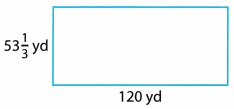a. What are the dimensions of a football field in feet?
There are 3 feet in 1 yard so 53$$\frac{1}{3}$$ = 53.$$\overline{3}$$ × 3 = 160 feet and 120 × 3 = 360 feet. The dimensions of the field are 120 by 360 feet.

b. A chalk line is placed around the perimeter of the football field. What is the length of this line in feet?
The length of this line in feet is 120 + 360 + 120 + 360 = 960 feet

c. About how many laps around the perimeter of the field would equal 1 mile? Explain.
There are 5280 feet in 1 mile so $$\frac{5280}{960}$$ = 5.5 laps around the field will be equal to 1 mile.

Question 21.
Look for a Pattern What is the result if you multiply a number of cups by $$\frac{8 \text { ounces }}{1 \text { cup }}$$ and then multiply the result by $$\frac{1 \text { cup }}{8 \text { ounces }}$$ ? Give an example.
For example, the number of cups is 8.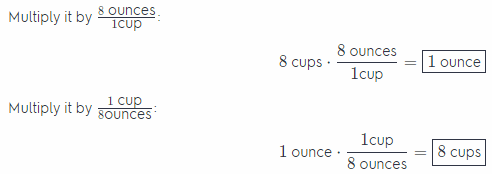The result is equal to the given number of cups.

For example, the number of cups is 16.The result is equal to the given number of cups.

Question 22.
Make a Conjecture 1 hour = 3,600 seconds and 1 mile = 5,280 feet. Make a conjecture about how you could convert a speed of 15 miles per hour to feet per second. Then convert.
The given rate is $$\frac{15}{1}$$, convert each dimension to required units, therefore: $$\frac{15}{1}=\frac{15 \times 5280}{1 \times 3600}$$ = 22 feet per second.